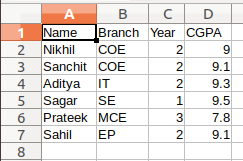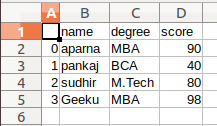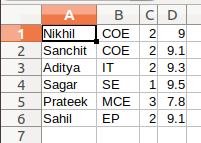Related Articles

# Python – Save List to CSV

• Difficulty Level : Basic
• Last Updated : 10 Jul, 2020

A CSV (Comma Separated Values) is a simple file format, used to store data in a tabular format. CSV file stores tabular data (numbers and text) in plain text. Each line of the file is a data record. Each record consists of one or more fields, separated by commas. The use of the comma as a field separator is the source of the name for this file format.

There are various methods to save lists to CSV which we will see in this article.

Method 1 : Using CSV Module

 `import` `csv`` ` ` ` `# field names ``fields ``=` `[``'Name'``, ``'Branch'``, ``'Year'``, ``'CGPA'``] ``   ` `# data rows of csv file ``rows ``=` `[ [``'Nikhil'``, ``'COE'``, ``'2'``, ``'9.0'``], ``         ``[``'Sanchit'``, ``'COE'``, ``'2'``, ``'9.1'``], ``         ``[``'Aditya'``, ``'IT'``, ``'2'``, ``'9.3'``], ``         ``[``'Sagar'``, ``'SE'``, ``'1'``, ``'9.5'``], ``         ``[``'Prateek'``, ``'MCE'``, ``'3'``, ``'7.8'``], ``         ``[``'Sahil'``, ``'EP'``, ``'2'``, ``'9.1'``]] `` ` `with ``open``(``'GFG'``, ``'w'``) as f:``     ` `    ``# using csv.writer method from CSV package``    ``write ``=` `csv.writer(f)``     ` `    ``write.writerow(fields)``    ``write.writerows(rows)`

Output:Method 2 : Using Pandas

 `# importing pandas as pd  ``import` `pandas as pd  `` ` `    ` `# list of name, degree, score ``nme ``=` `[``"aparna"``, ``"pankaj"``, ``"sudhir"``, ``"Geeku"``] ``deg ``=` `[``"MBA"``, ``"BCA"``, ``"M.Tech"``, ``"MBA"``] ``scr ``=` `[``90``, ``40``, ``80``, ``98``] ``    ` `# dictionary of lists  ``dict` `=` `{``'name'``: nme, ``'degree'``: deg, ``'score'``: scr}  ``      ` `df ``=` `pd.DataFrame(``dict``) ``   ` `# saving the dataframe ``df.to_csv(``'GFG.csv'``) `

Output:Method 3 : Using Numpy

 `import` `numpy as np`` ` ` ` `# data rows of csv file ``rows ``=` `[ [``'Nikhil'``, ``'COE'``, ``'2'``, ``'9.0'``], ``         ``[``'Sanchit'``, ``'COE'``, ``'2'``, ``'9.1'``], ``         ``[``'Aditya'``, ``'IT'``, ``'2'``, ``'9.3'``], ``         ``[``'Sagar'``, ``'SE'``, ``'1'``, ``'9.5'``], ``         ``[``'Prateek'``, ``'MCE'``, ``'3'``, ``'7.8'``], ``         ``[``'Sahil'``, ``'EP'``, ``'2'``, ``'9.1'``]] `` ` `# using the savetxt ``# from the numpy module``np.savetxt(``"GFG.csv"``, ``           ``rows,``           ``delimiter ``=``", "``, ``           ``fmt ``=``'% s'``)`

Output:Attention geek! Strengthen your foundations with the Python Programming Foundation Course and learn the basics.

To begin with, your interview preparations Enhance your Data Structures concepts with the Python DS Course. And to begin with your Machine Learning Journey, join the Machine Learning – Basic Level Course

My Personal Notes arrow_drop_up